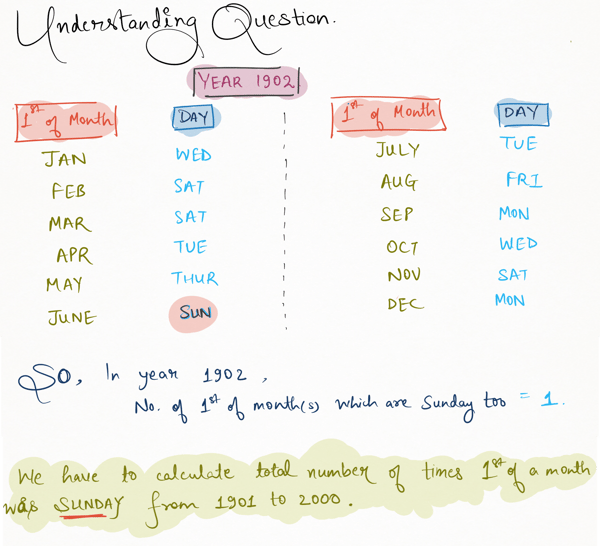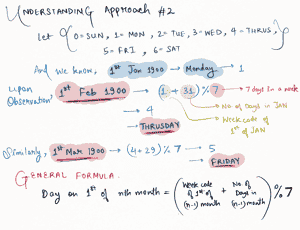Related Tags

python
communitycreator

# Project Euler 19

Armaan Nougai

### Counting Sundays

How many Sundays fell on the first of the month during the 20th century (1 January 1901 to 31 December 2000)?### Approach 1

In Python, if we create a datetime variable y then, y.strftime("%a") will return the weekday of the date.

 y=datetime(year,month,date)
weekday = y.strftime("%a")

• Using the function above, we can loop from 1901 to 2000, and for each year, we will check the 1st of every month.
• If we find the 1st of a month to be Sunday, we increment sunday_count by 1.
from datetime import datetime

sunday_count=0
for j in range(1901,2001):
for k in  range(1,13):
y = datetime(j,k,1)
if y.strftime("%a")=='Sun':
sunday_count+=1
print(sunday_count)


### Approach 2

A slightly better approach would be this.

Weekcode = { 0=Sunday, 1=Monday, 2=Tuesday, 3=Wednesday, 4=Thrusday, 5=Friday, 6=Saturday}


Generalized formula:

Weekcode on 1st of nth month of yth year = [ ( Weekcode on 1st of (n-1)th month of yth year ) + ( No. of Days in (n-1)th month ) ] % 7

• We make a for loop from 1900 to 2000 and for every year.
• We calculate the day on the 1st of each month by the generalized formula above.
• When we find the 1st of a month to be Sunday, we increment our sunday_count by 1.Weekcode = 1
# Weekcode of 1st Jan 1900 is i.e. Monday

months = [31,28,31,30,31,30,31,31,30,31,30,31]
# no. of days in each month ordered as they occurence in a year.

sunday_count = 0
# This is the sundays counter

for cur_year in range(1900,2000+1) :
# for loop from 1900 to 2000

# updating day count of Feb month according to the year
if (cur_year%4==0 and cur_year%100!=0) or (cur_year%400==0):
months = 29
else:
months = 28

for y in months:
Weekcode = (Weekcode + y)%7

if Weekcode==0 and cur_year>1900:
sunday_count+=1

print(sunday_count)

RELATED TAGS

python
communitycreator

CONTRIBUTOR

Armaan Nougai
RELATED COURSES

View all Courses

Keep Exploring

Learn in-demand tech skills in half the time